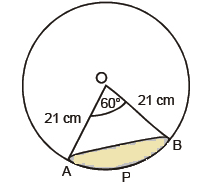Newbie

# In a circle of radius 21 cm, an arc subtends an angle of 60° at the centre. Find: (i) the length of the arc .Q.5(1)

• 0

Find the solution of the question 5(1) of exercise 12.2 of chapter areas related to circle ncert class 10 . Give me the easiest and simplest way to solve this question . Also find the best way to solve this question. In a circle of radius 21 cm, an arc subtends an angle of 60° at the centre. Find: (i) the length of the arc

Share

1. ution:Given,

Radius = 21 cm

θ = 60°

(i) Length of an arc = θ/360°×Circumference(2πr)

∴ Length of an arc AB = (60°/360°)×2×(22/7)×21

= (1/6)×2×(22/7)×21

Or Arc AB Length = 22cm

• 0